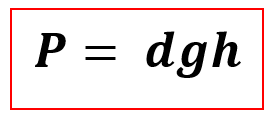# Problem: How tall must a water-filled manometer be to measure blood pressures as high as 294 mmHg? The densities of Mercury and Water are 13.6 g/cm3 and 1.00 g/cm3, respectively.

###### FREE Expert Solution

Here, we are asked to calculate the height of a water-filled manometer at a pressure of 294 mmHg. Given the densities of Mercury and Water.

• Mercury Density = 16.6 g/cm3
• Water Density= 1.00 g/cm3

The formula to be used is the Barometric Pressure formula:Where:

= pressure

= density of fluid (use the density of water since the manometer is water-filled)

= acceleration due to gravity

= height

79% (129 ratings)###### Problem Details

How tall must a water-filled manometer be to measure blood pressures as high as 294 mmHg? The densities of Mercury and Water are 13.6 g/cm3 and 1.00 g/cm3, respectively.